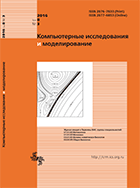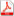Issue 3, 2016 Vol. 8

# All issues

Reduction of decision rule of multivariate interpolation and approximation method in the problem of data classificationpdf (539K)  / List of references

This article explores a method of machine learning based on the theory of random functions. One of the main problems of this method is that decision rule of a model becomes more complicated as the number of training dataset examples increases. The decision rule of the model is the most probable realization of a random function and it's represented as a polynomial with the number of terms equal to the number of training examples. In this article we will show the quick way of the number of training dataset examples reduction and, accordingly, the complexity of the decision rule. Reducing the number of examples of training dataset is due to the search and removal of weak elements that have little effect on the final form of the decision function, and noise sampling elements. For each $(x_i,y_i)$-th element sample was introduced the concept of value, which is expressed by the deviation of the estimated value of the decision function of the model at the point $x_i$, built without the $i$-th element, from the true value $y_i$. Also we show the possibility of indirect using weak elements in the process of training model without increasing the number of terms in the decision function. At the experimental part of the article, we show how changed amount of data affects to the ability of the method of generalizing in the classification task.

Keywords: machine learning, interpolation, approximation, random function, the system of linear equations, cross-validation, classification
Citation in English: Kopylov I.V. Reduction of decision rule of multivariate interpolation and approximation method in the problem of data classification // Computer Research and Modeling, 2016, vol. 8, no. 3, pp. 475-484
Citation in English: Kopylov I.V. Reduction of decision rule of multivariate interpolation and approximation method in the problem of data classification // Computer Research and Modeling, 2016, vol. 8, no. 3, pp. 475-484
DOI: 10.20537/2076-7633-2016-8-3-475-484
Views (last year): 5.

Full-text version of the journal is also available on the web site of the scientific electronic library eLIBRARY.RU

The journal is included in the Russian Science Citation Index

The journal is included in the List of Russian peer-reviewed journals publishing the main research results of PhD and doctoral dissertations.

International Interdisciplinary Conference "Mathematics. Computing. Education"

The journal is included in the RSCI

Indexed in Scopus